# >>> The Traveling Salesperson Problem¶

Consider the Traveling Salesperson Problem:

Given a set of cities and the distances between each pair of cities, what is the shortest possible tour that visits each city exactly once, and returns to the starting city?

In this notebook we will develop some solutions to the problem, and more generally show how to think about solving a problem like this.

## Understanding What We're Talking About (Vocabulary)¶

Do we understand precisely what the problem is asking? Do we understand all the concepts that the problem talks about? Do we understand them well enough to implement them in a programming language? Let's take a first pass:

• A set of cities: We will need to represent a set of cities; Python's set datatype might be appropriate.
• Distance between each pair of cities: If A and B are cities, this could be a function, distance(A, B), or a table lookup, distance[A][B]. The resulting distance will be a real number.
• City: All we have to know about an individual city is how far it is from other cities. We don't have to know its name, population, best restaurants, or anything else. So a city could be just an integer (0, 1, 2, ...) used as an index into a distance table, or a city could be a pair of (x, y) coordinates, if we are using straight-line distance on a plane.
• Tour: A tour is a specified order in which to visit the cities; Python's list or tuple datatypes would work. For example, given the set of cities {A, B, C, D}, a tour might be the list [B, D, A, C], which means to travel from B to D to A to C and finally back to B.
• Shortest possible tour: The shortest tour is the one whose tour length is the minimum of all tours.
• Tour length: The sum of the distances between adjacent cities in the tour (including the last city to the first city). Probably a function, tour_length(tour).
• What is ...: We can define a function to answer the question what is the shortest possible tour? The function takes a set of cities as input and returns a tour as output. I will use the convention that any such function will have a name ending in the letters "tsp", the traditional abbreviation for Traveling Salesperson Problem.

At this stage I have a rough sketch of how to attack the problem. I don't have all the answers, and I haven't committed to specific representations for all the concepts, but I know what all the pieces are, and I don't see anything that stops me from proceeding.

## Python and iPython Notebook: Preliminaries¶

This document is an iPython notebook: a mix of text and Python code that you can run. You can learn more from the "Help" button at the top of the page. But first we'll take care of some preliminaries: the Python statements that import standard libraries that we will be using later on:

In :
%pylab --no-import-all inline
from __future__ import division
import matplotlib.pyplot as plt
import random
import time
import itertools
import urllib
import csv

Populating the interactive namespace from numpy and matplotlib


# >>> All Tours Algorithm (alltours_tsp)¶

Let's start with an algorithm that is guaranteed to solve the problem (although it is inefficient for large sets of cities). Here is a sketch of the algorithm:

All Tours Algorithm: Generate all possible tours of the cities, and choose the shortest tour (the one with minimum tour length).

In general our design philosophy is to first write an English description of the algorithm, then write Python code that closely mirrors the English description. This will probably require some auxilliary functions and data structures; just assume they exist; put them on a TO DO list, and eventually define them with the same design philosophy.

Here is the start of the implementation:

In :
def alltours_tsp(cities):
"Generate all possible tours of the cities and choose the shortest tour."
return shortest_tour(alltours(cities))

def shortest_tour(tours):
"Choose the tour with the minimum tour length."
return min(tours, key=tour_length)

# TO DO: Data types: cities, tours, Functions: alltours, tour_length


Note: In Python min(collection,key=function) means to find the element x that is a member of collection such that function(x) is minimized. So shortest finds the tour whose tour_length in the minimal among the tours.

This gives us a good start; the Python code closely matches the English description. And we know what we need to do next: represent cities and tours, and implement the functions alltours and tour_length. Let's start with tours.

## Representing Tours¶

A tour starts in one city, and then visits each of the other cities in order, before returning to the start city. A natural representation of a tour is a sequence of cities. For example (1, 2, 3) could represent a tour that starts in city 1, moves to 2, then 3, and finally returns to 1.

Note: I considered using (1, 2, 3, 1) as the representation of this tour. I also considered an ordered list of edges between cities: ((1, 2), (2, 3), (3, 1)). In the end, I decided (1, 2, 3) was simplest.

Now for the alltours function. If a tour is a sequence of cities, then all the tours are permutations of the set of all cities. A function to generate all permutations of a set is already provided in Python's standard itertools library module; we can use it as our implementation of alltours:

In :
alltours = itertools.permutations


For n cities there are n! (that is, the factorial of n) permutations. Here's are all 3! = 6 tours of 3 cities:

In :
cities = {1, 2, 3}

list(alltours(cities))

Out:
[(1, 2, 3), (1, 3, 2), (2, 1, 3), (2, 3, 1), (3, 1, 2), (3, 2, 1)]

The length of a tour is the sum of the lengths of each edge in the tour; in other words, the sum of the distances between consecutive cities in the tour, including the distance form the last city back to the first:

In :
def tour_length(tour):
"The total of distances between each pair of consecutive cities in the tour."
return sum(distance(tour[i], tour[i-1])
for i in range(len(tour)))

# TO DO: Functions: distance, Data types: cities


Note: I use one Python-specific trick: when i is 0, then distance(tour, tour[-1]) gives us the wrap-around distance between the first and last cities, because tour[-1] is the last element of tour.

## Representing Cities¶

We determined that the only thing that matters about cities is the distance between them. But before we can decide about how to represent cities, and before we can define distance(A, B), we have to make a choice. In the fully general version of the TSP, the "distance" between two cities could be anything: it could factor in the amount of time it takes to travel between cities, the twistiness of the road, or anything else. The distance(A, B) might be different from distance(B, A). So the distances could be represented by a matrix distance[A][B], where any entry in the matrix could be any (non-negative) numeric value.

But we will ignore the fully general TSP and concentrate on an important special case, the Euclidean TSP, where the distance between any two cities is the Euclidean distance, the straight-line distance between points in a two-dimensional plane. So a city can be represented by a two-dimensional point: a pair of x and y coordinates. We will use the constructor function City, so that City(300, 0) creates a city with x-coordinate of 300 and y coordinate of 0. Then distance(A, B) will be a function that uses the x and y coordinates to compute the distance between A and B.

## Representing Points and Computing distance¶

OK, so a city can be represented as just a two-dimensional point. But how will we represent points? Here are some choices, with their pros and cons:

• tuple: A point is a two-tuple of (x, y) coordinates, for example, (300, 0). Pro: Very simple. Con: doesn't distinguish Points from other two-tuples.

• class: Define a custom Point class with x and y slots. Pro: explicit, gives us p.x and p.y accessors. Con: less efficient.

• complex: Python already has the two-dimensional point as a built-in numeric data type, but in a non-obvious way: as complex numbers, which inhabit the two-dimensional (real × imaginary) plane. Pro: efficient. Con: a little confusing; doesn't distinguish Points from other complex numbers.

Any of these choices would work perfectly well; I decided to use complex numbers and to implement the functions X and Y to make things less confusing. An advantage of complex numbers is that computing the distance between two points is easy—the absoute value of their difference:

In :
# Cities are represented as Points, which are represented as complex numbers
Point = complex
City  = Point

def X(point):
"The x coordinate of a point."
return point.real

def Y(point):
"The y coordinate of a point."
return point.imag

def distance(A, B):
"The distance between two points."
return abs(A - B)


Here's an example of computing the distance between two cities:

In :
A = City(3, 0)
B = City(0, 4)
distance(A, B)

Out:
5.0

## Random Sets of Cities¶

The input to a TSP algorithm should be a set of cities. I can make a random set of n cities by calling City n times, each with different random x and y coordinates:

In :
{City(random.randrange(1000), random.randrange(1000)) for c in range(6)}

Out:
{(288+9j), (668+743j), (817+11j), (669+414j), (810+496j), (659+568j)}

The function Cities does that (and a bit more):

In :
def Cities(n, width=900, height=600, seed=42):
"Make a set of n cities, each with random coordinates within a (width x height) rectangle."
random.seed(seed * n)
return frozenset(City(random.randrange(width), random.randrange(height))
for c in range(n))


There are three complications that I decided to tackle in Cities:

Complication 1: IPython's matplotlib plots (by default) in a rectangle that is 1.5 times wider than it is high; that's why I specified a width of 900 and a height of 600. If you want the coordinates of your cities to be bounded by a different size rectangle, you can change width or height.

Complication 2: Sometimes I want Cities(n) to be a true function, returning the same result each time. This is very helpful for getting repeatable results: if I run a test twice, I get the same results twice. But other times I would like to be able to do an experiment, where, for example, I call Cities(n) 30 times and get 30 different sets, and I then compute the average tour length produced by my algorithm across these 30 sets. Can I get both behaviors out of one function? Yes! The trick is the additional optional parameter, seed. Two calls to Cities with the same n and seed parameters will always return the same set of cities, and two calls with different values for seed will return different sets. This is implemented by calling the function random.seed, which resets the random number generator.

Complication 3: Once I create a set of Cities, I don't want anyone messing with my set. For example, I don't want an algorithm that claims to "solve" a problem by deleting half the cities from the input set, then finding a tour of the remaining cities. Therefore, I make Cities return a frozenset rather than a set. A frozenset is immutable; nobody can change it once it is created.

For example:

In :
# A set of 5 cities
Cities(5)

Out:
frozenset({(151+11j), (346+408j), (261+23j), (122+324j), (654+243j)})
In :
# The same set of 5 cities each time
[Cities(5) for i in range(3)]

Out:
[frozenset({(151+11j), (346+408j), (261+23j), (122+324j), (654+243j)}),
frozenset({(151+11j), (346+408j), (261+23j), (122+324j), (654+243j)}),
frozenset({(151+11j), (346+408j), (261+23j), (122+324j), (654+243j)})]
In :
# A different set of 5 cities each time
[Cities(5, seed=i) for i in range(3)]

Out:
[frozenset({(705+181j), (759+454j), (460+242j), (378+155j), (428+350j)}),
frozenset({(849+389j), (715+565j), (26+279j), (665+553j), (560+445j)}),
frozenset({(468+196j), (520+123j), (588+96j), (514+257j), (731+494j)})]

Now we are ready to apply the alltours_tsp function to find the shortest tour:

In :
alltours_tsp(Cities(8))

Out:
((648+296j),
(779+522j),
(329+588j),
(308+456j),
(5+449j),
(77+259j),
(715+107j),
(823+86j))
In :
tour_length(alltours_tsp(Cities(8)))

Out:
2395.20003959213

Quick, is that the right answer? I have no idea, and you probably can't tell either. But if we could plot the tour we'd understand it better and might be able to see at a glance if the tour is optimal.

## Plotting Tours¶

I define plot_tour(tour) to plot the cities (as circles) and the tour (as lines):

In :
def plot_tour(tour):
"Plot the cities as circles and the tour as lines between them."
plot_lines(list(tour) + [tour])

def plot_lines(points, style='bo-'):
"Plot lines to connect a series of points."
plt.plot(map(X, points), map(Y, points), style)
plt.axis('scaled'); plt.axis('off')

In :
plot_tour(alltours_tsp(Cities(8)))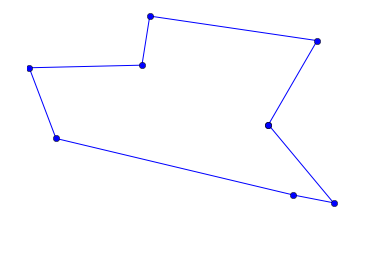That looks much better! To me, it looks like the shortest possible tour, although I don't have an easy way to prove it. Let's go one step further and define a function, plot_tsp(algorithm, cities) that will take a TSP algorithm (such as alltours_tsp) and a set of cities, apply the algorithm to the cities to get a tour, check that the tour is reasonable, plot the tour, and print information about the length of the tour and the time it took to find it:

In :
def plot_tsp(algorithm, cities):
"Apply a TSP algorithm to cities, plot the resulting tour, and print information."
# Find the solution and time how long it takes
t0 = time.clock()
tour = algorithm(cities)
t1 = time.clock()
assert valid_tour(tour, cities)
plot_tour(tour); plt.show()
print("{} city tour with length {:.1f} in {:.3f} secs for {}"
.format(len(tour), tour_length(tour), t1 - t0, algorithm.__name__))

def valid_tour(tour, cities):
"Is tour a valid tour for these cities?"
return set(tour) == set(cities) and len(tour) == len(cities)

In :
plot_tsp(alltours_tsp, Cities(8))8 city tour with length 2395.2 in 0.195 secs for alltours_tsp


## All Non-Redundant Tours Algorithm (improved alltours_tsp)¶

We said there are n! tours of n cities, and thus 6 tours of 3 cities:

In :
list(alltours({1, 2, 3}))

Out:
[(1, 2, 3), (1, 3, 2), (2, 1, 3), (2, 3, 1), (3, 1, 2), (3, 2, 1)]

But this is redundant: (1, 2, 3), (2, 3, 1), and (3, 1, 2) are three ways of describing the same tour. So let's arbitrarily say that all tours must start with the first city in the set of cities. We'll just pull the first city out, and then tack it back on to all the permutations of the rest of the cities.

While we're re-assembling a tour from the start city and the rest, we'll take the opportunity to construct the tour as a list rather than a tuple. It doesn't matter much now, but later on we will want to represent partial tours, to which we will want to append cities one by one; appending can only be done to lists, not tuples.

In :
def alltours(cities):
"Return a list of tours, each a permutation of cities, but each one starting with the same city."
start = first(cities)
return [[start] + Tour(rest)
for rest in itertools.permutations(cities - {start})]

def first(collection):
"Start iterating over collection, and return the first element."
return next(iter(collection))

Tour = list  # Tours are implemented as lists of cities


We can verify that for 3 cities there are now only 2 tours (not 6) and for 4 cities there are 6 tours (not 24):

In :
alltours({1, 2, 3})

Out:
[[1, 2, 3], [1, 3, 2]]
In :
alltours({1, 2, 3, 4})

Out:
[[1, 2, 3, 4],
[1, 2, 4, 3],
[1, 3, 2, 4],
[1, 3, 4, 2],
[1, 4, 2, 3],
[1, 4, 3, 2]]

Note: We could say that there is only one tour of three cities, because [1, 2, 3] and [1, 3, 2] are in some sense the same tour, one going clockwise and the other counterclockwise. However, I choose not to do that, for two reasons. First, it would mean we can never handle maps where the distance from A to B is different from B to A. Second, it would complicate the code (if only by a line or two) while not saving much run time.

We can verify that calling alltours_tsp(Cities(8)) still works and gives the same tour with the same total distance. But it now runs faster:

In :
plot_tsp(alltours_tsp, Cities(8))8 city tour with length 2395.2 in 0.051 secs for alltours_tsp


Now let's try a much harder 10-city tour:

In :
plot_tsp(alltours_tsp, Cities(10))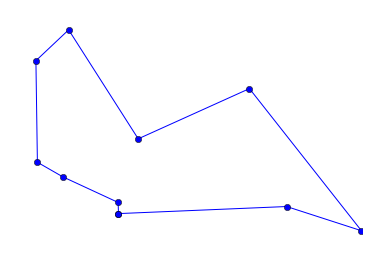10 city tour with length 2492.2 in 1.534 secs for alltours_tsp


## Complexity of alltours_tsp¶

It takes about 2 seconds on my machine to solve this 10-city problem. In general, the function TSP looks at (n-1)! tours for an n-city problem, and each tour has n cities, so the total time required for n cities should be roughly proportional to n!. This means that the time grows rapidly with the number of cities. Really rapidly. This table shows the actual time for solving a 10 city problem, and the exepcted time for solving larger problems:

n expected time for alltours_tsp(Cities(n))
10Covering 10! tours = 2 secs
112 secs × 11! / 10! ≈ 22 secs
122 secs × 12! / 10! ≈ 4 mins
142 secs × 14! / 10! ≈ 13 hours
162 secs × 16! / 10! ≈ 200 days
182 secs × 18! / 10! ≈ 112 years
252 secs × 25! / 10! ≈ 270 billion years

There must be a better way ...

# Approximate Algorithms¶

What if we are willing to settle for a tour that is short, but not guaranteed to be shortest? Then we can save billions of years of compute time: we will show several approximate algorithms, which find tours that are typically within 10% of the shortest possible tour, and can handle thousands of cities in a few seconds. (Note: There are more sophisticated approximate algorithms that can handle hundreds of thousands of cities and come within 0.01% or better of the shortest possible tour.)

So how do we come up with an approximate algorithm? Here are two general plans of how to create a tour:

• Nearest Neighbor Algorithm: Make the tour go from a city to its nearest neighbor. Repeat.
• Greedy Algorithm: Find the shortest distance between any two cities and include that edge in the tour. Repeat.

We will expand these ideas into full algorithms.

In addition, here are four very general strategies that apply not just to TSP, but to any optimization problem. An optimization problem is one in which the goal is to find a solution that is best (or near-best) according to some metric, out of a pool of many candidate solutions. The strategies are:

• Repetition Strategy: Take some algorithm and re-run it multiple times, varying some aspect each time, and take the solution with the best score.
• Alteration Strategy: Use some algorithm to create a solution, then make small changes to the solution to improve it.
• Ensemble Strategy: Take two or more algorithms, apply all of them to the problem, and pick the best solution.

And here are two more strategies that work for a wide variety of problems:

• Divide and Conquer: Split the input in half, solve the problem for each half, and then combine the two partial solutions.

• Stand on the Shoulders of Giants or Just Google It: Find out what others have done in the past, and either copy it or build on it.

# >>> Nearest Neighbor Algorithm (nn_tsp)¶

Here is a description of the nearest neighbor algorithm:

Nearest Neighbor Algorithm: Start at any city; at each step extend the tour by moving from the previous city to its nearest neighbor that has not yet been visited.

Often the best place for a tour to go next is to its nearest neighbor. But sometimes that neighbor would be better visited at some other point in the tour, so this algorithm is not guaranteed to find the shortest tour.

To implement the algorithm I need to represent all the noun phrases in the English description: "any city" (a city; arbitrarily the first city); "the tour" (a list of cities, initialy just the start city); "previous city" (the last element of tour, that is, tour[-1]); "nearest neighbor" (a function that, when given a city, A, and a list of other cities, finds the one with minimal distance from A); and "not yet been visited" (we will keep a set of unvisited cities; initially all cities but the start city are unvisited).

The algorithm (in more detail) is as follows:

• Keep track of a partial tour (initially just a single start city) and a list of unvisited cities
• While there are unvisited cities, do the following:
• find the unvisited city, C, that is nearest to the end city in the tour
• add C the end of the tour and remove it from the unvisited set
In :
def nn_tsp(cities):
"""Start the tour at the first city; at each step extend the tour
by moving from the previous city to its nearest neighbor
that has not yet been visited."""
start = first(cities)
tour = [start]
unvisited = set(cities - {start})
while unvisited:
C = nearest_neighbor(tour[-1], unvisited)
tour.append(C)
unvisited.remove(C)

def nearest_neighbor(A, cities):
"Find the city in cities that is nearest to city A."
return min(cities, key=lambda c: distance(c, A))


Note: In Python, as in the formal mathematical theory of computability, lambda (or λ) is the symbol for function, so "lambda c: distance(c, A)" means the function of c that computes the distance from c to the city A.

We can compare the fast (but approximate) nn_tsp algorithm to the slow (but optimal) alltours_tsp algorithm on a small map:

In :
plot_tsp(alltours_tsp, Cities(9))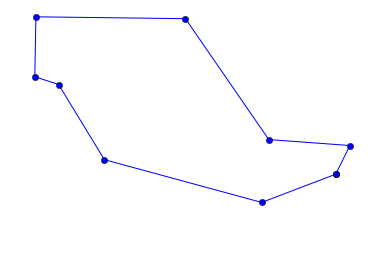9 city tour with length 2217.5 in 0.213 secs for alltours_tsp

In :
plot_tsp(nn_tsp, Cities(9))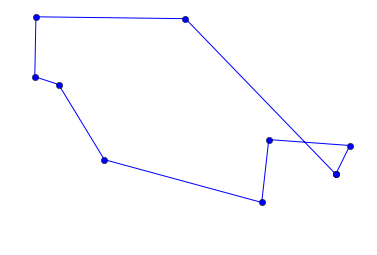9 city tour with length 2361.1 in 0.000 secs for nn_tsp


So the nearest neighbor approach was a lot faster, but it didn't find the shortest tour. To understand where it went wrong, it would be helpful to know what city it started from. I can modify plot_tour by adding one line of code to highlight the start city with a red square:

In :
def plot_tour(tour):
"Plot the cities as circles and the tour as lines between them. Start city is red square."
start = tour
plot_lines(list(tour) + [start])
plot_lines([start], 'rs') # Mark the start city with a red square

In :
plot_tsp(nn_tsp, Cities(9))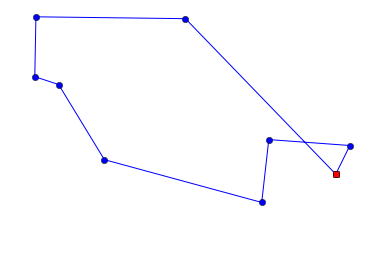9 city tour with length 2361.1 in 0.000 secs for nn_tsp


We can see that the tour moves clockwise from the start city, and mostly makes good decisions, but not optimal ones.

We can compare the performance of these two algorithms on, say, eleven different sets of cities instead of just one:

In :
def length_ratio(cities):
"The ratio of the tour lengths for nn_tsp and alltours_tsp algorithms."

sorted(length_ratio(Cities(8, seed=i)) for i in range(11))

Out:
[1.0,
1.0,
1.0,
1.0,
1.0,
1.0060657396717771,
1.0476876839109404,
1.061687777368538,
1.0980209876906022,
1.137595917252964,
1.1545257527154165]

The ratio of 1.0 means the two algorithms got the same (optimal) result; that happened 5 times out of 10. The other times, we see that the nn_tsp produces a longer tour, by anything up to 15% worse, with a median of 0.6% worse.

But more important than that 0.6% (or even 15%) difference is that the nearest neighbor algorithm can quickly tackle problems that the all tours algorithm can't touch in the lifetime of the universe. Finding a tour of 1000 cities takes well under a second:

In :
plot_tsp(nn_tsp, Cities(1000))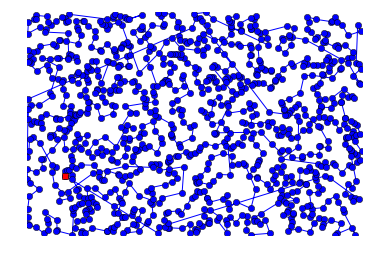1000 city tour with length 21523.4 in 0.217 secs for nn_tsp


Can we do better? Can we combine the speed of the nearest neighbor algorithm with the optimality of the all tours algorithm?

Let's consider what went wrong with nn_tsp. Looking at plot_tsp(nn_tsp, Cities(100)), we see that near the end of the tour there are some very long edges between cities, because there are no remaining cities near by. In a way, this just seems like bad luck—the way we flow from neighbor to neighbor just happens to leave a few very-long edges. Just as with buying lottery tickets, we could improve our chance of winning by trying more often; in other words, by using the repetition strategy.

## Repeated Nearest Neighbor Algorithm (repeated_nn_tsp)¶

Here is an easy way to apply the repetition strategy to improve nearest neighbors:

Repeated Nearest Neighbor Algorithm: For each of the cities, run the nearest neighbor algorithm with that city as the starting point, and choose the resulting tour with the shortest total distance.

So, with n cities we could run the nn_tsp algorithm n times, regrettably making the total run time n times longer, but hopefully making at least one of the n tours shorter.

To implement repeated_nn_tsp we just take the shortest tour over all starting cities:

In :
def repeated_nn_tsp(cities):
"Repeat the nn_tsp algorithm starting from each city; return the shortest tour."
return shortest_tour(nn_tsp(cities, start)
for start in cities)


To do that requires a modification of nn_tsp so that the start city can be specified as an optional argument:

In :
def nn_tsp(cities, start=None):
"""Start the tour at the first city; at each step extend the tour
by moving from the previous city to its nearest neighbor
that has not yet been visited."""
if start is None: start = first(cities)
tour = [start]
unvisited = set(cities - {start})
while unvisited:
C = nearest_neighbor(tour[-1], unvisited)
tour.append(C)
unvisited.remove(C)

In :
# Compare nn_tsp to repeated_nn_tsp
plot_tsp(nn_tsp, Cities(100))
plot_tsp(repeated_nn_tsp, Cities(100))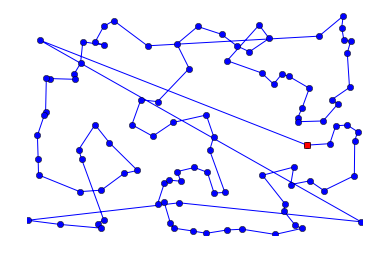100 city tour with length 8447.8 in 0.004 secs for nn_tsp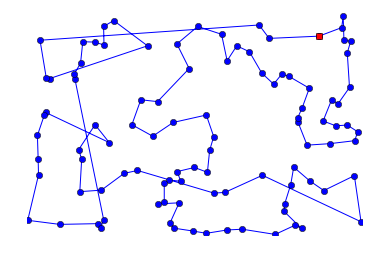100 city tour with length 6996.7 in 0.227 secs for repeated_nn_tsp


We see that repeated_nn_tsp does indeed take longer to run, and yields a tour that is shorter.

Let's try again with a smaller map that makes it easier to visualize the tours:

In :
plot_tsp(nn_tsp, Cities(9))
plot_tsp(repeated_nn_tsp, Cities(9))
plot_tsp(alltours_tsp, Cities(9))9 city tour with length 2361.1 in 0.000 secs for nn_tsp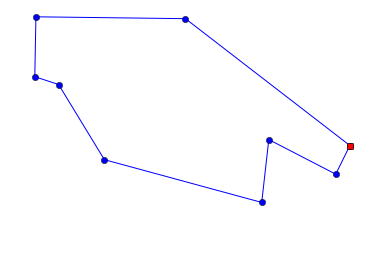9 city tour with length 2322.1 in 0.001 secs for repeated_nn_tsp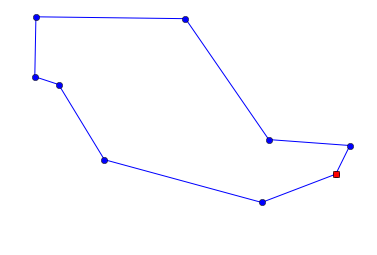9 city tour with length 2217.5 in 0.202 secs for alltours_tsp


This time the repeated_nn_tsp gives us a tour that is better tha nn_tsp, but not quite optimal. So, it looks like repetition is helping. But if I want to tackle 1000 cities, I don't really want the run time to be 1000 times slower. I'd like a way to moderate the repetition—to repeat the nn_tsp starting from a sample of the cities but not all the cities.

## Sampled Repeated Nearest Neighbor Algorithm (improved repeated_nn_tsp)¶

We can give repeated_nn_tsp an optional argument specifying the number of different cities to try starting from. We will implement the function sample to draw a random sample of the specified size from all the cities. Most of the work is done by the standard library function random.sample. What our sample adds is the same thing we did with the function Cities: we ensure that the function returns the same result each time for the same arguments, but can return different results if a seed parameter is passed in. (In addition, if the sample size, k is None or is larger than the population, then return the whole population.)

In :
def repeated_nn_tsp(cities, repetitions=100):
"Repeat the nn_tsp algorithm starting from specified number of cities; return the shortest tour."
return shortest_tour(nn_tsp(cities, start)
for start in sample(cities, repetitions))

def sample(population, k, seed=42):
"Return a list of k elements sampled from population. Set random.seed with seed."
if k is None or k > len(population):
return population
random.seed(len(population) * k * seed)
return random.sample(population, k)


Let's compare with 1, 10, and 100 starting cities on a 300 city map:

In :
def repeat_10_nn_tsp(cities): return repeated_nn_tsp(cities, 10)
def repeat_100_nn_tsp(cities): return repeated_nn_tsp(cities, 100)

In :
plot_tsp(nn_tsp, Cities(300))
plot_tsp(repeat_10_nn_tsp, Cities(300))
plot_tsp(repeat_100_nn_tsp, Cities(300))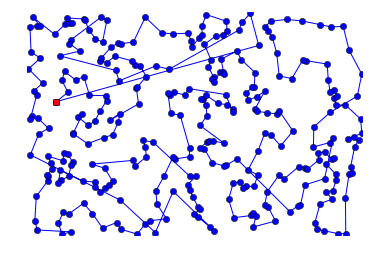300 city tour with length 11503.8 in 0.033 secs for nn_tsp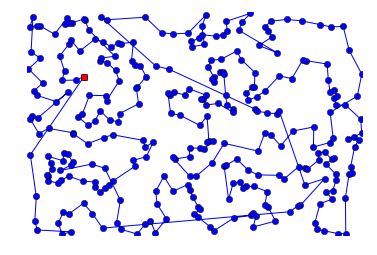300 city tour with length 11454.3 in 0.194 secs for repeat_10_nn_tsp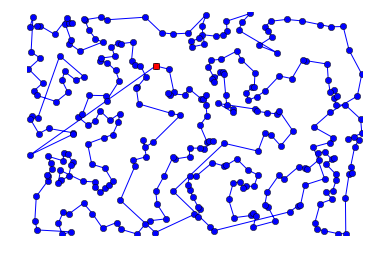300 city tour with length 11194.7 in 1.304 secs for repeat_100_nn_tsp


As we add more starting cities, the run times get longer and the tours get shorter.

I'd like to understand the tradefoff better. I'd like to have a way to compare different algorithms (or different choices of parameters for one algorithm) over multiple trials, and summarize the results. I'll get to that soon, but first a brief diversion for more vocabulary.

## New Vocabulary: Maps¶

We use the term cities and the function Cities to denote a set of cities. But now I want to talk about multiple trials over a collection of sets of cities: a plural of a plural. English doesn't give us a good way to do that, so it would be nice to have a singular noun that is a synonym for "set of cities." We'll use the term "map" for this, and the function Maps to create a collection of maps. Just like Cities, the function Maps will give the same result every time it is called with the same arguments.

In :
def Maps(num_maps, num_cities):
"Return a list of maps, each consisting of the given number of cities."
return tuple(Cities(num_cities, seed=(m, num_cities))
for m in range(num_maps))


## Benchmarking (Any Function)¶

The term benchmarking means running a function on a standard collection of inputs, in order to compare its performance. We'll define a general-purpose function, benchmark, which takes a function and a collection of inputs for that function, and runs the function on each of the inputs. It then returns two values: the average time taken per input, and the list of results of the function.

In :
def benchmark(function, inputs):
"Run function on all the inputs; return pair of (average_time_taken, results)."
t0           = time.clock()
results      = map(function, inputs)
t1           = time.clock()
average_time = (t1 - t0) / len(inputs)
return (average_time, results)


We can use benchmark to see that the abolute value function takes less than a microsecond:

In :
benchmark(abs, range(-10, 10))

Out:
(2.000000000279556e-07,
[10, 9, 8, 7, 6, 5, 4, 3, 2, 1, 0, 1, 2, 3, 4, 5, 6, 7, 8, 9])

And we can see that alltours_tsp can handle 6-city maps in about a millisecond each:

In :
benchmark(alltours_tsp, Maps(10, 6))

Out:
(0.0011380000000000834,
[[(654+277j), (508+326j), (208+535j), (188+480j), (156+351j), (595+162j)],
[(100+74j), (595+166j), (810+232j), (381+309j), (379+432j), (147+593j)],
[(502+121j), (380+210j), (237+277j), (272+467j), (531+557j), (889+424j)],
[(245+540j), (598+423j), (896+240j), (302+321j), (150+406j), (11+376j)],
[(862+280j), (710+441j), (652+440j), (405+598j), (362+42j), (755+142j)],
[(593+177j), (591+247j), (757+499j), (721+520j), (57+379j), (535+204j)],
[(253+77j), (242+298j), (34+377j), (283+545j), (529+364j), (827+8j)],
[(424+468j), (183+486j), (173+489j), (20+349j), (276+316j), (400+312j)],
[(669+590j), (790+529j), (507+186j), (80+41j), (240+328j), (505+564j)],
[(410+69j), (555+162j), (581+497j), (16+339j), (337+163j), (385+127j)]])

## Caching (or Memoization) of Function Results¶

Here's an issue about benchmarking: each time we develop a new algorithm, we would like to compare its performance to some standard old algorithms. It seems a waste of computer time to re-run the old algorithms on a standard data set each time. And it seems like a waste of human time to scroll back up and check (e.g. "How did nn_tsp do on Maps(30, 60)? Where was that?"). It would be better if the benchmark function could remember the (function, inputs) pairs that it has already run, and when asked for the same pair over again, just go look up the results rather than re-computing the results.

The process of taking a function (such as benchmark) and making it remember its previously-computed results is called memoization. The idea is that we do:

benchmark = memoize(benchmark)



and now when we call benchmark(function, test_cases) the first time it will be computed just like before, but when we make the same call a second time, the result will be instanly looked up rather than laboriously re-computed.

Here is one way to implement the function memoized so that memoized(func) creates a new function that acts just like func except that it stores results in a table, called a cache:

In :
def memoize(func):
"Return a version of func that remembers the output for each input."
cache = {}
def new_func(*args):
try:
# If we have already computed func(*args), just return it
return cache[args]
except TypeError:
# If args are not hashable, compute func(*args) and return it
return func(*args)
except KeyError:
# If args are hashable, compute func(*args) and store it
cache[args] = func(*args)
return cache[args]
new_func._cache   = cache
new_func.__doc__  = func.__doc__
new_func.__name__ = func.__name__
return new_func


There are three paths through the function:

1. If the tuple args is already in the cache, then look it up and return it.
2. A TypeError means that one of the args is not hashable; in that case we just call func(*args) and return it. We can't do caching in this case.
3. A KeyError means that args are hashable, but have not yet been seen. So compute func(*args), store the result in the cache, and return it.

We also copy over the documentation and function name from the old func to the new memoized function, and store the cache there as well, so that, if we want to to, we could do benchmark._cache.clear().

We could now do "benchmark = memoize(benchmark)", or more Pythonically, use the decorator syntax:

In :
@memoize
def benchmark(function, inputs):
"Run function on all the inputs; return pair of (average_time_taken, results)."
t0           = time.clock()
results      = map(function, inputs)
t1           = time.clock()
average_time = (t1 - t0) / len(inputs)
return (average_time, results)


Here we see that the first call to benchmark takes 5 seconds, while the second repeated call takes only microseconds but correctly delivers the same result:

In :
%time benchmark(alltours_tsp, Maps(2, 10))

CPU times: user 3.22 s, sys: 65.8 ms, total: 3.28 s
Wall time: 3.22 s

Out:
(1.6421194999999997,
[[(664+505j),
(617+543j),
(500+564j),
(57+539j),
(98+220j),
(797+56j),
(781+126j),
(583+264j),
(485+435j),
(587+492j)],
[(560+80j),
(743+218j),
(461+142j),
(420+268j),
(153+264j),
(152+251j),
(297+73j),
(363+53j),
(434+55j),
(482+20j)]])
In :
%time benchmark(alltours_tsp, Maps(2, 10))

CPU times: user 86 µs, sys: 27 µs, total: 113 µs
Wall time: 98.9 µs

Out:
(1.6421194999999997,
[[(664+505j),
(617+543j),
(500+564j),
(57+539j),
(98+220j),
(797+56j),
(781+126j),
(583+264j),
(485+435j),
(587+492j)],
[(560+80j),
(743+218j),
(461+142j),
(420+268j),
(153+264j),
(152+251j),
(297+73j),
(363+53j),
(434+55j),
(482+20j)]])

## Benchmarking (TSP Algorithms)¶

Now let's add another function, benchmarks, which builds on benchmark in two ways:

1. It compares multiple algorithms, rather than just running one algorithm. (Hence the plural benchmarks.)
2. It is specific to TSP algorithms, and rather than returning results, it prints summary statistics: the mean, standard deviation, min, and max of tour lengths, as well as the time taken and the number and size of the sets of cities.
In :
def benchmarks(tsp_algorithms, maps=Maps(30, 60)):
"Print benchmark statistics for each of the algorithms."
for tsp in tsp_algorithms:
time, results = benchmark(tsp, maps)
lengths = map(tour_length, results)
print("{:>25} |{:7.1f} ±{:4.0f} ({:5.0f} to {:5.0f}) |{:7.3f} secs/map | {} ⨉ {}-city maps"
.format(tsp.__name__, mean(lengths), stddev(lengths), min(lengths), max(lengths),
time, len(maps), len(maps)))


Here are two functions to do simple statistics:

In :
def mean(numbers): return sum(numbers) / len(numbers)

def stddev(numbers):
"The standard deviation of a collection of numbers."
return (mean([x ** 2 for x in numbers]) - mean(numbers) ** 2) ** 0.5


## How Many Starting Cities is best for nn_tsp?¶

Now we are in a position to gain some insight into how many repetitions, or starting cities, we need to get a good result from nn_tsp.

In :
def repeat_25_nn_tsp(cities): return repeated_nn_tsp(cities, 25)
def repeat_50_nn_tsp(cities): return repeated_nn_tsp(cities, 50)

In :
algorithms = [nn_tsp, repeat_10_nn_tsp, repeat_25_nn_tsp, repeat_50_nn_tsp, repeat_100_nn_tsp]

benchmarks(algorithms)

                   nn_tsp | 5689.8 ± 487 ( 4536 to  6861) |  0.001 secs/map | 30 ⨉ 60-city maps
repeat_10_nn_tsp | 5238.1 ± 396 ( 4150 to  5977) |  0.006 secs/map | 30 ⨉ 60-city maps
repeat_25_nn_tsp | 5137.2 ± 375 ( 4071 to  6181) |  0.014 secs/map | 30 ⨉ 60-city maps
repeat_50_nn_tsp | 5123.1 ± 358 ( 4071 to  5977) |  0.029 secs/map | 30 ⨉ 60-city maps
repeat_100_nn_tsp | 5116.0 ± 352 ( 4071 to  5977) |  0.033 secs/map | 30 ⨉ 60-city maps


We see that adding more starting cities results in shorter tours, but you start getting diminishing returns after 25 or 50 repetitions.

Let's try again with bigger maps:

In :
benchmarks(algorithms, Maps(30, 120))

                   nn_tsp | 7843.8 ± 457 ( 6993 to  8983) |  0.005 secs/map | 30 ⨉ 120-city maps
repeat_10_nn_tsp | 7361.4 ± 365 ( 6824 to  8157) |  0.021 secs/map | 30 ⨉ 120-city maps
repeat_25_nn_tsp | 7249.5 ± 353 ( 6527 to  7984) |  0.052 secs/map | 30 ⨉ 120-city maps
repeat_50_nn_tsp | 7230.6 ± 336 ( 6647 to  7969) |  0.123 secs/map | 30 ⨉ 120-city maps
repeat_100_nn_tsp | 7201.4 ± 345 ( 6527 to  7957) |  0.283 secs/map | 30 ⨉ 120-city maps

In :
benchmarks(algorithms, Maps(30, 150))

                   nn_tsp | 8657.3 ± 417 ( 7743 to  9323) |  0.006 secs/map | 30 ⨉ 150-city maps
repeat_10_nn_tsp | 8268.5 ± 357 ( 7637 to  9260) |  0.040 secs/map | 30 ⨉ 150-city maps
repeat_25_nn_tsp | 8168.0 ± 380 ( 7429 to  9369) |  0.099 secs/map | 30 ⨉ 150-city maps
repeat_50_nn_tsp | 8091.9 ± 349 ( 7511 to  9250) |  0.199 secs/map | 30 ⨉ 150-city maps
repeat_100_nn_tsp | 8067.2 ± 351 ( 7409 to  9238) |  0.399 secs/map | 30 ⨉ 150-city maps


The results are similar. So depending on what your priorities are (run time versus tour length), somewhere around 25 or 50 repetitions might be a good tradeoff.

Next let's try to analyze where nearest neighbors goes wrong, and see if we can do something about it.

## A Problem with Nearest Neighbors: Outliers¶

Consider the 20-city map that we build below:

In :
outliers_list = [City(2, 2),  City(2, 3),  City(2, 4),  City(2, 5),  City(2, 6),
City(3, 6),  City(4, 6),  City(5, 6),  City(6, 6),
City(6, 5),  City(6, 4),  City(6, 3),  City(6, 2),
City(5, 2),  City(4, 2),  City(3, 2),
City(1, 6.8),  City(7.8, 6.4),  City(7, 1.2),  City(0.2, 2.8)]

outliers = set(outliers_list)

plot_lines(outliers, 'bo')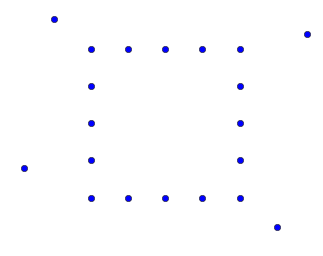Let's see what a nearest neighbor search does on this map:

In :
plot_tsp(nn_tsp, outliers)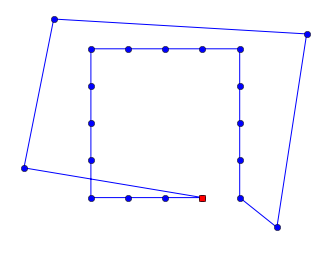20 city tour with length 37.3 in 0.000 secs for nn_tsp


The tour starts out going around the inner square. But then we are left with four long lines to pick up the outliers. Let's try to understand what went wrong. First we'll create a new tool to draw better diagrams:

In :
def plot_labeled_lines(points, *args):
"""Plot individual points, labeled with an index number.
Then, args describe lines to draw between those points.
An arg can be a matplotlib style, like 'ro--', which sets the style until changed,
or it can be a list of indexes of points, like [0, 1, 2], saying what line to draw."""
# Draw points and label them with their index number
plot_lines(points, 'bo')
for (label, p) in enumerate(points):
plt.text(X(p), Y(p), '  '+str(label))
# Draw lines indicated by args
style = 'bo-'
for arg in args:
if isinstance(arg, str):
style = arg
else: # arg is a list of indexes into points, forming a line
Xs = [X(points[i]) for i in arg]
Ys = [Y(points[i]) for i in arg]
plt.plot(Xs, Ys, style)
plt.axis('scaled'); plt.axis('off'); plt.show()


In the diagram below, imagine we are running a nearest neighbor algorithm, and it has created a partial tour from city 0 to city 4. Now there is a choice. City 5 is the nearest neighbor. But if we don't take city 16 at this point, we will have to pay a higher price sometime later to pick up city 16.

In :
plot_labeled_lines(outliers_list, 'bo-', [0, 1, 2, 3, 4], 'ro--', [4, 16], 'bo--', [4, 5])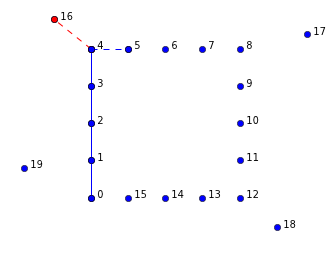It seems that picking up an outlier is sometimes a good idea, but sometimes going directly to the nearest neighbor is a better idea. So what can we do? It is difficult to make the choice between an outlier and a nearest neighbor while we are constructing a tour, because we don't have the context of the whole tour yet. So here's an alternative idea: don't try to make the right choice while constructing the tour; just go ahead and make any choice, then when the tour is complete, alter it to correct problems caused by outliers (or other causes).

## New Vocabulary: Segment¶

We'll define a segment as a subsequence of a tour: a sequence of consecutive cities within a tour. A tour forms a loop, but a segment does not have a loop; it is open-ended on both ends. So, if [A, B, C, D] is a 4-city tour, then segments include [A, B], [B, C, D], and many others. Note that the segment [A, B, C, D] is different than the tour [A, B, C, D]; the tour returns from D to A but the segment does not.

# >>> Altering Tours by Reversing Segments¶

One way we could try to improve a tour is by reversing a segment. Consider this tour:

In :
cross = [City(9, 3),  City(3, 10),  City(2, 16),  City(3, 21),  City(9, 28),
City(26, 3), City(32, 10), City(33, 16), City(32, 21), City(26, 28)]

plot_labeled_lines(cross, range(-1,10))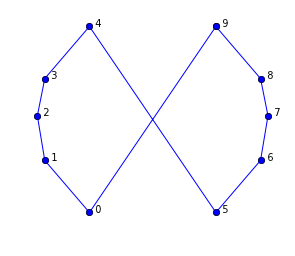This is clearly not an optimal tour. We should "uncross" the lines, which can be achieved by reversing a segment. The tour as it stands is [0, 1, 2, 3, 4, 5, 6, 7, 8, 9]. If we reverse the segment [5, 6, 7, 8, 9], we get the tour [0, 1, 2, 3, 4, 9, 8, 7, 6, 5], which is the optimal tour. In the diagram below, reversing [5, 6, 7, 8, 9] is equivalent to deleting the red dashed lines and adding the green dotted lines. If the sum of the lengths of the green dotted lines is less than the sum of the lengths of the red dashed lines, then we know the reversal is an improvement.

In :
plot_labeled_lines(cross, 'bo-', range(5), range(5, 10),
'g:', (4, 9), (0, 5),
'r--', (4, 5), (0, 9))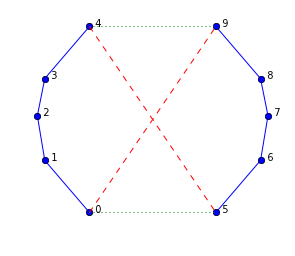Here we see that reversing [5, 6, 7, 8, 9] works:

In :
tour = Tour(cross)
tour[5:10] = reversed(tour[5:10])
plot_tour(tour)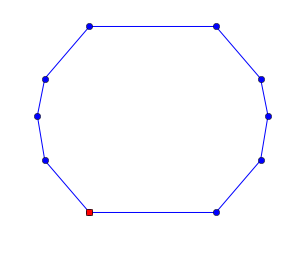Here is how we can check if reversing a segment is an improvement, and if so to do it:

In :
def reverse_segment_if_better(tour, i, j):
"If reversing tour[i:j] would make the tour shorter, then do it."
# Given tour [...A-B...C-D...], consider reversing B...C to get [...A-C...B-D...]
A, B, C, D = tour[i-1], tour[i], tour[j-1], tour[j % len(tour)]
# Are old edges (AB + CD) longer than new ones (AC + BD)? If so, reverse segment.
if distance(A, B) + distance(C, D) > distance(A, C) + distance(B, D):
tour[i:j] = reversed(tour[i:j])


Now let's write a function, alter_tour, which finds segments to swap. What segments should we consider? I don't know how to be clever about the choice, but I do know how to be fairly thorough: try all segments of all lengths at all starting positions. I have an intuition that trying longer ones first is better (although I'm not sure).

I worry that even trying all segements won't be enough: after I reverse one segment, it might open up opportunities to reverse other segments. So, after trying all possible segments, I'll check the tour length. If it has been reduced, I'll go through the alter_tour process again.

In :
def alter_tour(tour):
"Try to alter tour for the better by reversing segments."
original_length = tour_length(tour)
for (start, end) in all_segments(len(tour)):
reverse_segment_if_better(tour, start, end)
if tour_length(tour) < original_length:
return alter_tour(tour)

def all_segments(N):
"Return (start, end) pairs of indexes that form segments of tour of length N."
return [(start, start + length)
for length in range(N, 2-1, -1)
for start in range(N - length + 1)]


Here is what the list of all segments look like, for N=4:

In :
all_segments(4)

Out:
[(0, 4), (0, 3), (1, 4), (0, 2), (1, 3), (2, 4)]

We can see that altering the cross tour does straighten it out:

In :
plot_tour(alter_tour(Tour(cross)))## Altered Nearest Neighbor Algorithm (altered_nn_tsp)¶

Let's see what happens when we alter the output of nn_tsp:

In :
def altered_nn_tsp(cities):
"Run nearest neighbor TSP algorithm, and alter the results by reversing segments."
return alter_tour(nn_tsp(cities))


Let's try this new algorithm on some test cases:

In :
plot_tsp(altered_nn_tsp, set(cross))10 city tour with length 93.2 in 0.000 secs for altered_nn_tsp

In :
plot_tsp(altered_nn_tsp, Cities(9))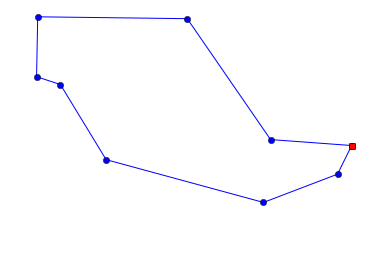9 city tour with length 2217.5 in 0.000 secs for altered_nn_tsp


It gets the optimal results. Let's try benchmarking:

In :
algorithms = [nn_tsp, repeat_50_nn_tsp, altered_nn_tsp]

benchmarks(algorithms)

                   nn_tsp | 5689.8 ± 487 ( 4536 to  6861) |  0.001 secs/map | 30 ⨉ 60-city maps
repeat_50_nn_tsp | 5123.1 ± 358 ( 4071 to  5977) |  0.041 secs/map | 30 ⨉ 60-city maps
altered_nn_tsp | 4812.1 ± 263 ( 4091 to  5320) |  0.012 secs/map | 30 ⨉ 60-city maps


This is quite encouraging; altered_nn_tsp gives shorter tours and is three times faster than repeating nearest neighbors from 50 starting cities. Could we do better?

## Altered Repeated Nearest Neighbor Algorithm (altered_repeated_nn_tsp)¶

We have seen that the nearest neighbor algorithm is improved by both the alteration and repetition strategies. So why not apply both strategies?

In :
def repeated_altered_nn_tsp(cities, repetitions=20):
"Use alteration to improve each repetition of nearest neighbors."
return shortest_tour(alter_tour(nn_tsp(cities, start))
for start in sample(cities, repetitions))

def repeat_5_altered_nn_tsp(cities): return repeated_altered_nn_tsp(cities, 5)


Let's see it in action:

In :
plot_tsp(repeated_altered_nn_tsp, Cities(100))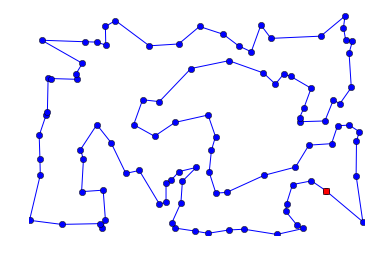100 city tour with length 5851.6 in 0.831 secs for repeated_altered_nn_tsp


That looks like a good tour. Let's gather more data:

In :
algorithms = [nn_tsp, repeat_50_nn_tsp, altered_nn_tsp, repeated_altered_nn_tsp]

benchmarks(algorithms)
print('-' * 100)
benchmarks(algorithms, Maps(30, 120))

                   nn_tsp | 5689.8 ± 487 ( 4536 to  6861) |  0.001 secs/map | 30 ⨉ 60-city maps
repeat_50_nn_tsp | 5123.1 ± 358 ( 4071 to  5977) |  0.041 secs/map | 30 ⨉ 60-city maps
altered_nn_tsp | 4812.1 ± 263 ( 4091 to  5320) |  0.012 secs/map | 30 ⨉ 60-city maps
repeated_altered_nn_tsp | 4628.8 ± 233 ( 3925 to  5120) |  0.238 secs/map | 30 ⨉ 60-city maps
----------------------------------------------------------------------------------------------------
nn_tsp | 7843.8 ± 457 ( 6993 to  8983) |  0.004 secs/map | 30 ⨉ 120-city maps
repeat_50_nn_tsp | 7230.6 ± 336 ( 6647 to  7969) |  0.154 secs/map | 30 ⨉ 120-city maps
altered_nn_tsp | 6622.8 ± 261 ( 6064 to  7155) |  0.054 secs/map | 30 ⨉ 120-city maps
repeated_altered_nn_tsp | 6441.1 ± 215 ( 6000 to  6946) |  1.124 secs/map | 30 ⨉ 120-city maps


So, repetition gives us a modest improvement in tour length over the altered_nn_tsp, at the cost of 20 times more run time. It looks like the alteration strategy is more powerful than the repetition strategy in this case.

# Non-Random Maps¶

I thought it would be fun to work on some real maps, instead of random maps. First I found a page that lists geographical coordinates of US cities. Here is an excerpt from that page:

[TCL]  33.23   87.62  Tuscaloosa,AL
[FLG]  35.13  111.67  Flagstaff,AZ
[PHX]  33.43  112.02  Phoenix,AZ


I also found a blog post by Randal S. Olson who chose 50 landmarks across the states and found a tour based on actual road-travel distances, not straight-line distance. His data looks like this:

Mount Rushmore National Memorial, South Dakota 244, Keystone, SD    43.879102   -103.459067
Toltec Mounds, Scott, AR    34.647037   -92.065143
Ashfall Fossil Bed, Royal, NE   42.425000   -98.158611

You can't see, but fields are separated by tabs in this data.

Now we have a problem: we have two similar but different data formats, and we want to convert both of them to Maps (sets of cities). Python provides a module, csv (for "comma-separated values"), to parse data like this. The function csv.reader takes an input that should be an iterable over lines of text, and optionally you can tell it what character to use as a delimiter (as well as several other options). For each line, it generates a list of fields. For example, for the line "[TCL] 33.23 87.62 Tuscaloosa,AL" it would generate the list ['[TCL]', '33.23', '87.62', 'Tuscaloosa,AL'].

I define the function Coordinate_map to take an iterable of lines (usually a file object or a list of strings), parse it with csv_reader, pick out the latitude and longitude columns, and build a City out of each one:

In :
def lines(text): return text.strip().splitlines()

def Coordinate_map(lines, delimiter=' ', lat_col=1, long_col=2, lat_scale=69, long_scale=-48):
"""Make a set of Cities from an iterable of lines of text.
Specify the column delimiter, and the zero-based column number of lat and long.
Treat long/lat as a square x/y grid, scaled by long_scale and lat_scale.
Source can be a file object, or list of lines."""
return frozenset(City(long_scale * float(row[long_col]),
lat_scale  * float(row[lat_col]))
for row in csv.reader(lines, delimiter=delimiter, skipinitialspace=True))


You might be wondering about the lat_scale=69, long_scale=-48 part. The issue is that we have latitude and longitude for cities, and we want to compute the distance between cities. To do that accurately requires complicated trigonometry. But we can get an approximation by assuming the earth is flat, and that latitude and longitude are on a rectangular grid. (This is a bad approximation if you're talking about distances of 10,000 miles, but close enough for 100 miles, as long as you're not too close to the poles.) I took the latitude of the center of the country (Wichita, KS: latitude 37.65) and plugged it into a Length Of A Degree Of Latitude And Longitude Calculator to find that, in Wichita, one degree of latitude is 69 miles, and one degree of longitude is 48 miles. (It is -48 rather than +48 because the US is west of the prime meridian.)

Now let's create the map of USA cities, and find a tour for it:

In :
USA_map = Coordinate_map(lines("""
[TCL]  33.23   87.62  Tuscaloosa,AL
[FLG]  35.13  111.67  Flagstaff,AZ
[PHX]  33.43  112.02  Phoenix,AZ
[PGA]  36.93  111.45  Page,AZ
[TUS]  32.12  110.93  Tucson,AZ
[LIT]  35.22   92.38  Little Rock,AR
[SFO]  37.62  122.38  San Francisco,CA
[LAX]  33.93  118.40  Los Angeles,CA
[SAC]  38.52  121.50  Sacramento,CA
[SAN]  32.73  117.17  San Diego,CA
[SBP]  35.23  120.65  San Luis Obi,CA
[EKA]  41.33  124.28  Eureka,CA
[DEN]  39.75  104.87  Denver,CO
[DCA]  38.85   77.04  Washington/Natl,DC
[MIA]  25.82   80.28  Miami Intl,FL
[TPA]  27.97   82.53  Tampa Intl,FL
[JAX]  30.50   81.70  Jacksonville,FL
[TLH]  30.38   84.37  Tallahassee,FL
[ATL]  33.65   84.42  Atlanta,GA
[BOI]  43.57  116.22  Boise,ID
[CHI]  41.90   87.65  Chicago,IL
[IND]  39.73   86.27  Indianapolis,IN
[DSM]  41.53   93.65  Des Moines,IA
[SUX]  42.40   96.38  Sioux City,IA
[ICT]  37.65   97.43  Wichita,KS
[LEX]  38.05   85.00  Lexington,KY
[NEW]  30.03   90.03  New Orleans,LA
[BOS]  42.37   71.03  Boston,MA
[PWM]  43.65   70.32  Portland,ME
[BGR]  44.80   68.82  Bangor,ME
[CAR]  46.87   68.02  Caribou Mun,ME
[DET]  42.42   83.02  Detroit,MI
[STC]  45.55   94.07  St Cloud,MN
[DLH]  46.83   92.18  Duluth,MN
[STL]  38.75   90.37  St Louis,MO
[JAN]  32.32   90.08  Jackson,MS
[BIL]  45.80  108.53  Billings,MT
[BTM]  45.95  112.50  Butte,MT
[RDU]  35.87   78.78  Raleigh-Durh,NC
[INT]  36.13   80.23  Winston-Salem,NC
[OMA]  41.30   95.90  Omaha/Eppley,NE
[LAS]  36.08  115.17  Las Vegas,NV
[RNO]  39.50  119.78  Reno,NV
[AWH]  41.33  116.25  Wildhorse,NV
[EWR]  40.70   74.17  Newark Intl,NJ
[SAF]  35.62  106.08  Santa Fe,NM
[NYC]  40.77   73.98  New York,NY
[BUF]  42.93   78.73  Buffalo,NY
[ALB]  42.75   73.80  Albany,NY
[FAR]  46.90   96.80  Fargo,ND
[BIS]  46.77  100.75  Bismarck,ND
[CVG]  39.05   84.67  Cincinnati,OH
[CLE]  41.42   81.87  Cleveland,OH
[OKC]  35.40   97.60  Oklahoma Cty,OK
[PDX]  45.60  122.60  Portland,OR
[MFR]  42.37  122.87  Medford,OR
[AGC]  40.35   79.93  Pittsburgh,PA
[PVD]  41.73   71.43  Providence,RI
[CHS]  32.90   80.03  Charleston,SC
[RAP]  44.05  103.07  Rapid City,SD
[FSD]  43.58   96.73  Sioux Falls,SD
[MEM]  35.05   90.00  Memphis Intl,TN
[TYS]  35.82   83.98  Knoxville,TN
[CRP]  27.77   97.50  Corpus Chrst,TX
[DRT]  29.37  100.92  Del Rio,TX
[IAH]  29.97   95.35  Houston,TX
[SAT]  29.53   98.47  San Antonio,TX
[LGU]  41.78  111.85  Logan,UT
[SLC]  40.78  111.97  Salt Lake Ct,UT
[SGU]  37.08  113.60  Saint George,UT
[CNY]  38.77  109.75  Moab,UT
[MPV]  44.20   72.57  Montpelier,VT
[RIC]  37.50   77.33  Richmond,VA
[BLI]  48.80  122.53  Bellingham,WA
[SEA]  47.45  122.30  Seattle,WA
[ALW]  46.10  118.28  Walla Walla,WA
[GRB]  44.48   88.13  Green Bay,WI
[MKE]  42.95   87.90  Milwaukee,WI
[CYS]  41.15  104.82  Cheyenne,WY
[SHR]  44.77  106.97  Sheridan,WY
"""))

In :
plot_lines(USA_map, 'bo')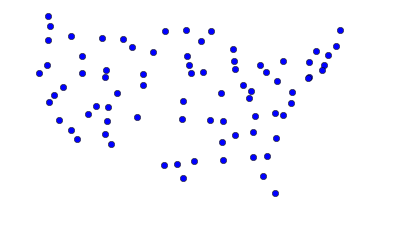In :
plot_tsp(repeated_altered_nn_tsp, USA_map)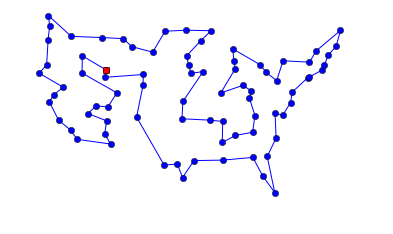80 city tour with length 13633.0 in 0.471 secs for repeated_altered_nn_tsp


Not bad! There are no obvious errors in the tour (although I'm not at all confident it is the optimal tour).

Now let's do the same for Randal Olson's landmarks. Note that the data is delimited by tabs, not spaces, and the longitude already has a minus sign, so we don't need another one in long_scale.

In :
USA_landmarks_map = Coordinate_map(lines("""
Mount Rushmore National Memorial, South Dakota 244, Keystone, SD	43.879102	-103.459067
Toltec Mounds, Scott, AR	34.647037	-92.065143
Ashfall Fossil Bed, Royal, NE	42.425000	-98.158611
Maryland State House, 100 State Cir, Annapolis, MD 21401	38.978828	-76.490974
The Mark Twain House & Museum, Farmington Avenue, Hartford, CT	41.766759	-72.701173
Columbia River Gorge National Scenic Area, Oregon	45.711564	-121.519633
Mammoth Cave National Park, Mammoth Cave Pkwy, Mammoth Cave, KY	37.186998	-86.100528
Bryce Canyon National Park, Hwy 63, Bryce, UT	37.593038	-112.187089
USS Alabama, Battleship Parkway, Mobile, AL	30.681803	-88.014426
Graceland, Elvis Presley Boulevard, Memphis, TN	35.047691	-90.026049
Wright Brothers National Memorial Visitor Center, Manteo, NC	35.908226	-75.675730
Vicksburg National Military Park, Clay Street, Vicksburg, MS	32.346550	-90.849850
Statue of Liberty, Liberty Island, NYC, NY	40.689249	-74.044500
Mount Vernon, Fairfax County, Virginia	38.729314	-77.107386
Fort Union Trading Post National Historic Site, Williston, North Dakota 1804, ND	48.000160	-104.041483
San Andreas Fault, San Benito County, CA	36.576088	-120.987632
Chickasaw National Recreation Area, 1008 W 2nd St, Sulphur, OK 73086	34.457043	-97.012213
Hanford Site, Benton County, WA	46.550684	-119.488974
Spring Grove Cemetery, Spring Grove Avenue, Cincinnati, OH	39.174331	-84.524997
Craters of the Moon National Monument & Preserve, Arco, ID	43.416650	-113.516650
The Alamo, Alamo Plaza, San Antonio, TX	29.425967	-98.486142
New Castle Historic District, Delaware	38.910832	-75.527670
Gateway Arch, Washington Avenue, St Louis, MO	38.624647	-90.184992
Okefenokee Swamp Park, Okefenokee Swamp Park Road, Waycross, GA	31.056794	-82.272327
Cape Canaveral, FL	28.388333	-80.603611
Glacier National Park, West Glacier, MT	48.759613	-113.787023
Congress Hall, Congress Place, Cape May, NJ 08204	38.931843	-74.924184
Olympia Entertainment, Woodward Avenue, Detroit, MI	42.387579	-83.084943
Fort Snelling, Tower Avenue, Saint Paul, MN	44.892850	-93.180627
Hoover Dam, Boulder City, CO	36.012638	-114.742225
White House, Pennsylvania Avenue Northwest, Washington, DC	38.897676	-77.036530
USS Constitution, Boston, MA	42.372470	-71.056575
Omni Mount Washington Resort, Mount Washington Hotel Road, Bretton Woods, NH	44.258120	-71.441189
Grand Canyon National Park, Arizona	36.106965	-112.112997
The Breakers, Ochre Point Avenue, Newport, RI	41.469858	-71.298265
Fort Sumter National Monument, Sullivan's Island, SC	32.752348	-79.874692
Cable Car Museum, 94108, 1201 Mason St, San Francisco, CA 94108	37.794781	-122.411715
Yellowstone National Park, WY 82190	44.462085	-110.642441
French Quarter, New Orleans, LA	29.958443	-90.064411
C. W. Parker Carousel Museum, South Esplanade Street, Leavenworth, KS	39.317245	-94.909536
Shelburne Farms, Harbor Road, Shelburne, VT	44.408948	-73.247227
Taliesin, County Road C, Spring Green, Wisconsin	43.141031	-90.070467
Acadia National Park, Maine	44.338556	-68.273335
Liberty Bell, 6th Street, Philadelphia, PA	39.949610	-75.150282
Terrace Hill, Grand Avenue, Des Moines, IA	41.583218	-93.648542
Lincoln Home National Historic Site Visitor Center, 426 South 7th Street, Springfield, IL	39.797501	-89.646211
Lost World Caverns, Lewisburg, WV	37.801788	-80.445630
"""), delimiter='\t', long_scale=48)

In :
plot_lines(USA_landmarks_map, 'bo')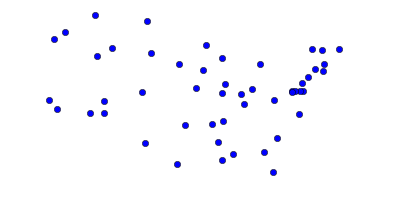In :
plot_tsp(repeated_altered_nn_tsp, USA_landmarks_map)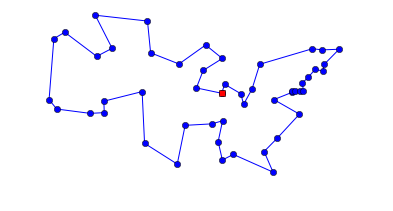50 city tour with length 10235.3 in 0.205 secs for repeated_altered_nn_tsp


We can compare that to the tour that Randal Olson computed as the shortest based on road distances: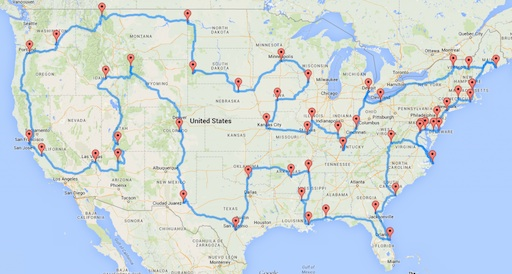The two tours are similar but not the same. I think the difference is that roads through the rockies and along the coast of the Carolinas tend to be very windy, so Randal's tour avoids them, whereas my straight-line tour does not. William Cook provides an analysis, and a tour that is shorter than either Randal's or mine.

Now let's go back to the original web page to get a bigger map with over 1000 cities. I note that the page has some lines that I don't want, so I will filter out lines that are not in the continental US (that is, cities in Alaska or Hawaii), as well as header lines that do not start with '['.

In :
url = 'http://www.realestate3d.com/gps/latlong.htm'

def continental_USA(line):
"Does line denote a city in the continental United States?"
return line.startswith('[') and ',AK' not in line and ',HI' not in line

USA_big_map = Coordinate_map(filter(continental_USA, urllib.urlopen(url)))

In :
plot_lines(USA_big_map, 'bo')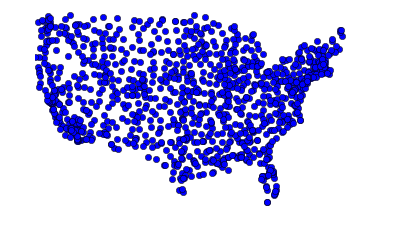Let's get a baseline tour with nn_tsp:

In :
plot_tsp(nn_tsp, USA_big_map)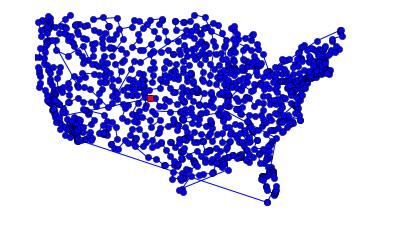1089 city tour with length 52919.1 in 0.297 secs for nn_tsp


Now try to improve on that with repeat_100_nn_tsp and with repeat_5_altered_nn_tsp (which will take a while with over 1000 cities):

In :
plot_tsp(repeat_100_nn_tsp, USA_big_map)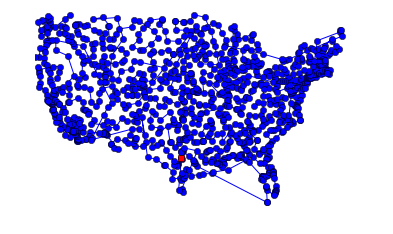1089 city tour with length 51166.0 in 24.777 secs for repeat_100_nn_tsp

In :
plot_tsp(repeat_5_altered_nn_tsp, USA_big_map)